### Home > PC > Chapter 6 > Lesson 6.1.2 > Problem6-31

6-31.
1. . Use the Law of Cosines or Law of Sines to find the value of x in each triangle below. REMEMBER TO CHANGE TO DEGREE MODE! Homework Help ✎

1.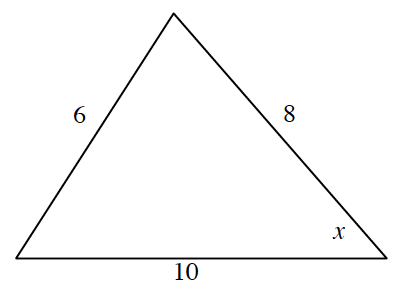2.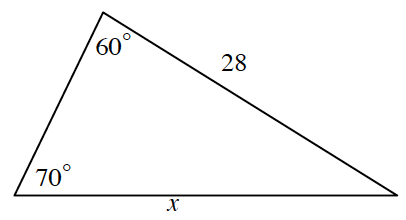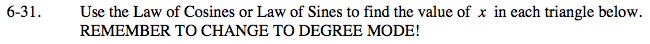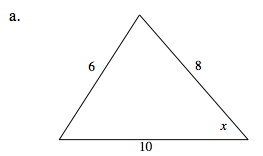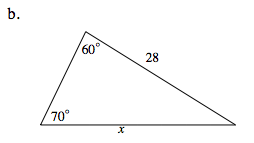Law of Sines

$\frac{a}{\text{sin}A}=\frac{b}{\text{sin}B}=\frac{c}{\text{sin}C}$

Law of Cosines

a2 = b2 + c2 − 2bcCos(A)